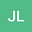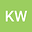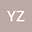Calculation and analysis of the strong prism of the octagonal-quadrilateral networks
•••Kang Wang
Anhui Jianzhu University South Campus
Author ProfileYa-Qian Zheng
Anhui Jianzhu University
Author Profile## Abstract

Nowadays, with the development of the times, network structure analysis has become a hot issue in some fields. The eigenvalues of normalized Laplacian are very important for some network structure properties. Let Qn be octagonal-quadrilateral networks composed of n octagons and n squares and let Q2n be the strong prism of Qn. The strong product of a complete graph of order 2 and a complete graph of order G forms a strong prism of the graph G. In this paper, the decomposition theorem of the associated matrix is used to completely investigate the normalized Laplacian spectrum of Qn2. In addition, we establish exact formulas for the degree-Kirchhoff index and the number of spanning trees of Qn2.